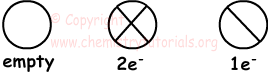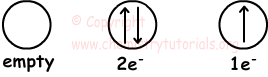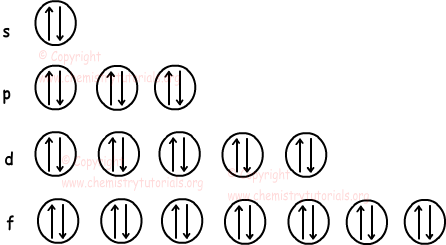Menu

## Atomic Structure Cheatsheet

Atomic Structure Cheat Sheet

Atomic Structure

Atom is the smallest particle of matter. It consist of three particles, called proton, electron and neutron. Protons and neutrons are placed at the center of the atom and electrons are placed around the center.

Protons: Protons are positively charged particles of atoms. They are located in nucleus, and have mass. We show protons with letter "p".

Electrons: Electrons are negatively charged moving particles of atoms. They are located around the nucleus on orbits, and have no mass. We show electrons with letter "e".

Neutrons: Neutrons are charge-less particles of atoms. They are located in nucleus, and have mass. We show neutrons with letter "n".

Atomic Number:

Atomic number shows the number of protons in each atom. It is specific for each atom and shown with letter "Z".

Mass Number:

Mass number of atom is equal to the sum of mass of protons and neutrons.

M=n+p

We show M on atom as;

ZMX

Isotopes:

Isotopes have same atomic number but different mass number.

Isotones:

Isotone atoms have same  number of neutrons and different numbers of protons.

Isobars:

Isobar atoms have different atomic number and different neutron number but same mass number.

Electron Configuration

Electrons are not placed at fixed positions in atoms, but we can predict approximate positions of them. These positions are called energy levels or shells of atoms.

• Lowest energy level is 1 and it is denoted with integer n=1, 2, 3, 4, 5, 6... or letters starting from K, L, N to Q.  An atom can have maximum 7 energy levels and electrons can change their levels according to their energies.
• Each energy level has different number of electrons. For example, we can find number of electrons in four energy level with following formula; 2n2.

Showing Electrons in Orbitals (sub shells)

We show orbitals with following shape;Capacity of each orbital is two electrons. We can also show electrons in orbitals as follows ;There are some rules for placing electrons in orbitals.Look at the following figure that shows number of orbitals in each sub levels s, p, d, f;Spherical Symmetry

Spherical symmetry makes atom more stable. Half filled or filled orbitals show us spherical symmetry.

s : 1e- or 2e-

p : 3e- or 6e-

d : 5e- or 10e-

f: 7e- or 14e-

Orbitals s, p, d, f have given electrons above, has spherical symmetry.

Electron Configurations of Ions

If  electron is bound to neutral atom, atom becomes negatively charged ion. We calculate total number of electrons and make electron configuration.

Atomic Structure Exams and  Problem Solutions

Author:

© Copyright www.ChemistryTutorials.org, Reproduction in electronic and written form is expressly forbidden without written permission of www.ChemistryTutorials.org. Privacy Policy